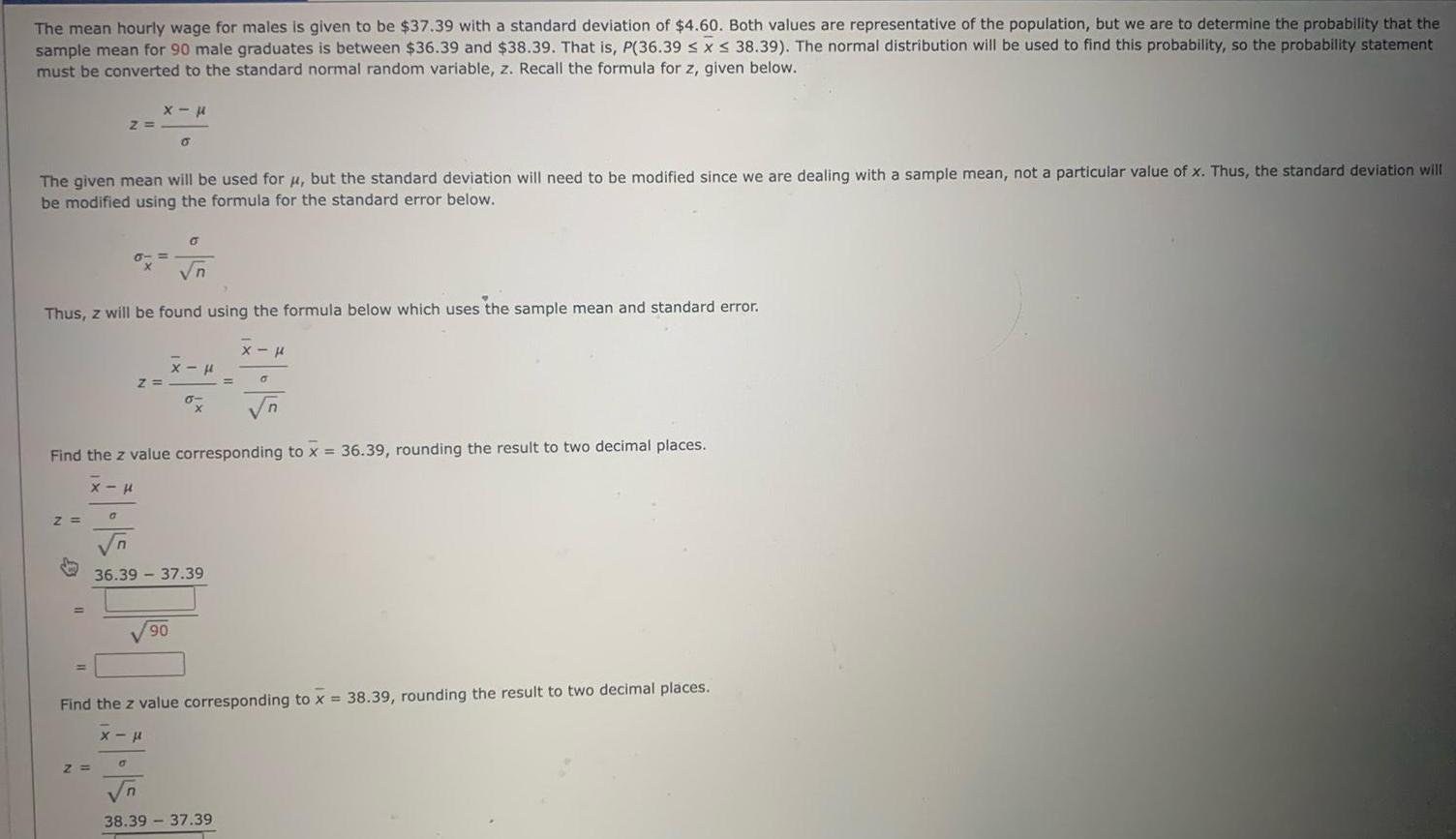Question:

# The mean hourly wage for males is given to be 37 39 with a

Last updated: 11/21/2023The mean hourly wage for males is given to be 37 39 with a standard deviation of 4 60 Both values are representative of the population but we are to determine the probability that the sample mean for 90 male graduates is between 36 39 and 38 39 That is P 36 39 x 38 39 The normal distribution will be used to find this probability so the probability statement must be converted to the standard normal random variable z Recall the formula for z given below The given mean will be used for but the standard deviation will need to be modified since we are dealing with a sample mean not a particular value of x Thus the standard deviation will be modified using the formula for the standard error below Z Z 0 Z n Thus z will be found using the formula below which uses the sample mean and standard error n x Z a 6 0 Find the z value corresponding to x 36 39 rounding the result to two decimal places x 90 x H 36 39 37 39 6 x x Find the z value corresponding to x 38 39 rounding the result to two decimal places x n 38 39 37 39 0 n